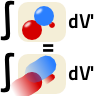Lorentz invariance is at the core of any (special) relativistic theory. Sometimes, however, we encounter expressions that seem to transform in a wrong way. This is especially true for the four-potential calculated from the retarded four-current. Find out why the four-potential is indeed Lorentz invariant!

## Problem Statement

The well-known retarded integrations over the charge density ρ(xν) and current density j(xν)\give rise to the scalar and vecor potential:

$\begin{eqnarray*} \phi\left(x^{\nu}\right)&=&\frac{1}{4\pi\varepsilon_{0}} \int \frac{1}{\left|\mathbf{r}-\mathbf{r}^{\prime} \right|}\rho \left(\mathbf{r}^{\prime},t- \left|\mathbf{r}-\mathbf{r}^{\prime} \right|/c\right) dV^{\prime}\ \text{and}\\ \mathbf{A}\left(x^{\nu}\right)& = &\frac{\mu_{0}}{4\pi}\int \frac{1}{\left|\mathbf{r}- \mathbf{r}^{\prime}\right|} \mathbf{j}\left(\mathbf{r}^{\prime},t-\left|\mathbf{r} -\mathbf{r}^{\prime} \right|/c\right)dV^{\prime}\ . \end{eqnarray*}$

At a first glance, these equations seem to be not Lorentz invariant. Show that the four-potential $$A^{\mu}\left(x^{\nu}\right)=\left(\phi\left(x^{\nu}\right)/c,\,\mathbf{A}\left(x^{\nu}\right)\right)$$ obeys indeed Lorentz invariance:

Express Aµ in terms of the four-current jµ,

Re-forumlate the integral to go over space and time, $$dV^{\prime}\rightarrow dV^{\prime}dt^{\prime}$$ using the δ-distribution,

$\text{use}\quad \delta\left[f\left(x\right)\right] = \sum_{\text{roots }x_{i}} \frac{\delta\left(x-x_{i}\right)}{\left|f^{\prime} \left(x_{i}\right)\right|}$

to find a forumulation of the integral without the $$1/\left|\mathbf{r}-\mathbf{r}^{\prime}\right|$$.

## Hints

Remember that we use SI units in which

$j^{\mu}\left(x^{\nu}\right)=\left(c \rho\left(x^{\nu}\right),\mathbf{j} \left(x^{\nu}\right)\right)$

For the last part of the problem: Think of the variables of the argument of the δ-distribution (a function!) such that the derivative of it is $$\propto \left|\mathbf{r}-\mathbf{r}^{\prime} \right|$$.

Let us find the implications of the Lorentz invariance of the four-potential!

## Four-Potential and Four-Current

Let us first write down how we calculate the four-potential for a given coordinate system

$x^{\mu}=\left(ct,\mathbf{r}\right)$

with respect to some charges and currents. In the necessary retarded formulation we have

$A^{\mu}\left(x^{\nu}\right) = \left(\begin{array}{c}\phi\left(x^{\nu} \right)/c\\ \mathbf{A}\left(x^{\nu}\right) \end{array}\right) = \frac{1}{4\pi} \int\frac{1}{ \left|\mathbf{r}- \mathbf{r}^{\prime}\right|} \left(\begin{array}{c} \rho \left(\mathbf{r}^{\prime},t- \left|\mathbf{r}-\mathbf{r}^{\prime}\right|/c\right)/ \varepsilon_{0}c\\ \mu_{0}\mathbf{j} \left(\mathbf{r}^{\prime}, t-\left|\mathbf{r}- \mathbf{r}^{\prime} \right|/c\right) \end{array}\right) dV^{\prime}\ .$

Now we can remember that

$j^{\mu} \left(x^{\nu}\right)=\left(c\rho\left(x^{\nu}\right), \mathbf{j} \left(x^{\nu}\right)\right)$

and conclude:

$A^{\mu}\left(x^{\nu}\right) = \frac{\mu_{0}}{4\pi}\int\frac{j^{\mu} \left(\mathbf{r}^{\prime},t- \left|\mathbf{r}- \mathbf{r}^{\prime} \right|/c\right)}{\left|\mathbf{r}-\mathbf{r}^{\prime} \right|} dV^{\prime}\ ,$

since

$\varepsilon_{0}\mu_{0} \equiv1/c^{2}\text{ and thus }1/ \varepsilon_{0}c=\mu_{0}c$

This result is of course nice but we probably knew it already as solution to $$\Box A^{\mu}=j^{\mu}$$.

Nevertheless, the integral does not seem to be Lorentz invariant at first sight. So, what can we do to show it?

## Reformulation of the Integral

We can try to bring the integral into a form such that it's an integral with invariant quantities each, i.e. no retardation. Ok, we may simply write

$A^{\mu}\left(x^{\nu}\right) = \frac{\mu_{0}}{4\pi}\int\frac{j^{\mu} \left(\mathbf{r}^{\prime}, t^{\prime}\right)}{\left|\mathbf{r}- \mathbf{r}^{\prime}\right|} \delta\left(c\left(t-t^{\prime}\right)- \left|\mathbf{r}- \mathbf{r}^{\prime}\right|\right) \theta\left(t-t^{\prime}\right) dV^{\prime}dt^{\prime}$

and keep in mind that t > t' since we only care about the retarted interaction, not the advanced one. This fact is reflected with the Heaviside-theta and will be important later on.

Now we want to get rid of anything looking non-Lorentz-invariant. The only chance we have is try something with the δ-distribution - maybe we can get rid of the distance |r - r'|!

## δ-Gymnastics and Lorentz-Invariance

Ok, let us use

$\delta\left[f\left(x\right)\right] = \sum_{\text{roots }x_{i}}\frac{\delta\left(x-x_{i}\right)}{\left|f^{\prime}\left(x_{i}\right)\right|}$

to re-formulate the expression inside the δ-distribution regarding it at a fixed spacial distance  |r - r'| and with variable τ = c(t - t'):

$\begin{eqnarray*} \delta \left[\left(x^{\nu}-x^{\nu\prime}\right)^{2}\right]& = &\delta\left[-\tau^{2}+\left|\mathbf{r}- \mathbf{r}^{\prime}\right|\right]\\& = &\delta\left[\left(-\tau+\left|\mathbf{r}-\mathbf{r}^{\prime}\right|\right)\left(\tau+\left|\mathbf{r} -\mathbf{r}^{\prime}\right|\right)\right]\\& = &\frac{1}{2\left|\mathbf{r}- \mathbf{r}^{\prime}\right|}\left[\delta\left(-\tau+\left|\mathbf{r}- \mathbf{r}^{\prime}\right|\right)+ \delta\left(\tau+\left|\mathbf{r}-\mathbf{r}^{\prime}\right|\right)\right]\ . \end{eqnarray*}$

Then, we end up with

$A^{\mu}\left(x^{\nu}\right) = \frac{\mu_{0}}{2\pi}\int j^{\mu}\left(x^{\nu\prime}\right)\delta \left(\left(x^{\nu}-x^{\nu\prime} \right)^{2}\right) \theta\left(t-t^{\prime}\right)dV^{\prime}dt^{\prime}\ .$

Note that the decomposition of the δ-distribution resulted in two contributions- retarded, with "-τ" and advanced, with "+τ". Luckily, the θ-distribution fixes the issue. The result is astonishing since we end up with just an integration over the four-current which looks somehow unfamiliar.

The given integral is naturally invariant since any quantity involved is Lorentz-invariant: the integral measure, the current and

$\left(x^{\nu}-x^{\nu\prime} \right)^{2}=\eta_{\mu\nu} x^{\mu}x^{\nu\prime}$